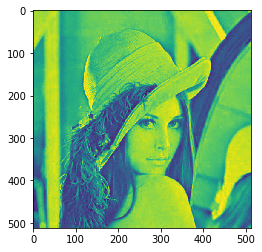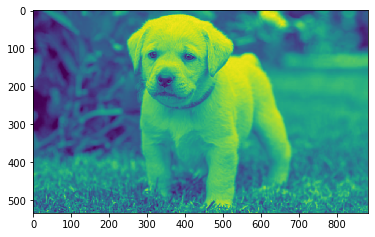# Mahotas – Getting Image Moments

• Last Updated : 29 May, 2021

In this article we will see how we can the image moments in mahotas. In image processing, computer vision and related fields, an image moment is a certain particular weighted average of the image pixels’ intensities, or a function of such moments, usually chosen to have some attractive property or interpretation. Image moments are useful to describe objects after segmentation.

In this tutorial we will use “lena” image, below is the command to load it.

Attention geek! Strengthen your foundations with the Python Programming Foundation Course and learn the basics.

To begin with, your interview preparations Enhance your Data Structures concepts with the Python DS Course. And to begin with your Machine Learning Journey, join the Machine Learning - Basic Level Course

`mahotas.demos.load('lena')`

Below is the lena imageIn order to do this we will use mahotas.moments method
Syntax : mahotas.moments(img, p0, p1)
Argument : It takes image object and two float values as argument
Return : It returns image object

Note : Input image should be filtered or should be loaded as grey
In order to filter the image we will take the image object which is numpy.ndarray and filter it with the help of indexing, below is the command to do this

`image = image[:, :, 0]`

Below is the implementation

## Python3

 `# importing required libraries``import` `mahotas``import` `mahotas.demos``from` `pylab ``import` `gray, imshow, show``import` `numpy as np``import` `matplotlib.pyplot as plt``  ` `# loading image``img ``=` `mahotas.demos.load(``'lena'``)``  ` `# filtering image``img ``=` `img.``max``(``2``)` `print``(``"Image"``)``  ` `# showing image``imshow(img)``show()` `# Power for first dimension``p0 ``=` `5.5` `# Power for second dimension``p1 ``=` `5.5`  `# getting moments``moment ``=` `mahotas.moments(img, p0, p1)`` `  `# printing moments``print``(``"Moment value = "` `+` `str``(moment))`

Output :

```Image
````Moment value = 6.784986531904299e+35`

Another example

## Python3

 `# importing required libraries``import` `mahotas``import` `numpy as np``from` `pylab ``import` `gray, imshow, show``import` `os``import` `matplotlib.pyplot as plt`` ` `# loading image``img ``=` `mahotas.imread(``'dog_image.png'``)`  `# filtering image``img ``=` `img[:, :, ``0``]``  ` `print``(``"Image"``)``  ` `# showing image``imshow(img)``show()` `# Power for first dimension``p0 ``=` `10.5` `# Power for second dimension``p1 ``=` `2.5`  `# getting moments``moment ``=` `mahotas.moments(img, p0, p1)`` `  `# printing moments``print``(``"Moment value = "` `+` `str``(moment))`

Output :

```Image
````Moment value = 1.5229432312149368e+42`

My Personal Notes arrow_drop_up